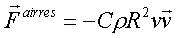Teaching Physics with the Physics Suite Edward F. Redish

Air Resistance 3: Deriving the equation

In this problem, you will derive an explicit form of Newton's drag law for air resistance whose structure we derived in a previous problem by dimensional analysis. The derivation below will provide the dimensionless coefficient that we were unable to find by dimensional analysis.

(a) Consider a small particle of mass m. (Ignore gravity.) The particle is approached by a very massive wall moving toward it with a velocity v. After the wall hits it, what velocity will the small particle have? (Hint: Consider first the case of the small particle moving toward the wall with a velocity -v. Solve what happens; then hop onto a moving frame.)

(b) Suppose the moving wall is a disk of radius R moving at a velocity v in a direction perpendicular to the plane of the disk. If there are N small particles per unit volume in the region of space the disk is sweeping through, how many of them will it encounter in a small time Dt?

(c) Calculate the total momentum transferred to the air in the time Dt by the disk assuming that there are N air particles per unit volume and they each have mass m.

(d) Find the force the disk exerts on the air and the force the air exerts on the disk. How do you know?

(e) Show that the force you calculated has the formand find the dimensionless constant, C.

Not finding what you wanted? Check the Site Map for more information.